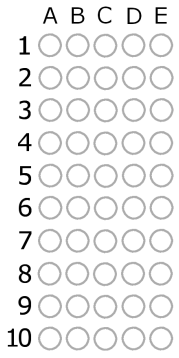top of page

## Day 66 - Lesson 5.3

##### Learning Targets
• Determine whether or not a given scenario is a binomial setting.

• Calculate probabilities involving a single value of a binomial random variable.

• Make a histogram to display a binomial distribution and describe its shape.

##### Activity:

We had a little fun with the kids today.  We told them to put everything away but a pencil for a… POP QUIZ!!!  Students, of course, began panicking. We passed out the activity page and told them to mark their answers for the ten questions.  The kicker: they did not get to read the questions!  At this point the kids caught on and let out a sigh.

Students worked through the remainder of the activity with little problem since they had already seen the binomial theorem during the Three Shots activity.  They used the applet to create a histogram.They can use the histogram to approximate the probability of guessing exactly 4 questions correct.  They will then calculate the exact probability of guessing exactly 4 out of 10 correct using the binomial theorem.

##### Teaching Tip:

By doing the Three Shots activity first, students should have a good conceptual understanding of the binomial theorem.  Consider using the same language as you did during Three Shots: Success=Make and Failure=Miss.  When working through a binomial problem, we will often ask, “How many makes? What’s the probability? How many misses? What’s the probability?”  Students get really used to this pattern and find binomial probability very easy, which no one would expect when you look at the formula the first time!

bottom of page